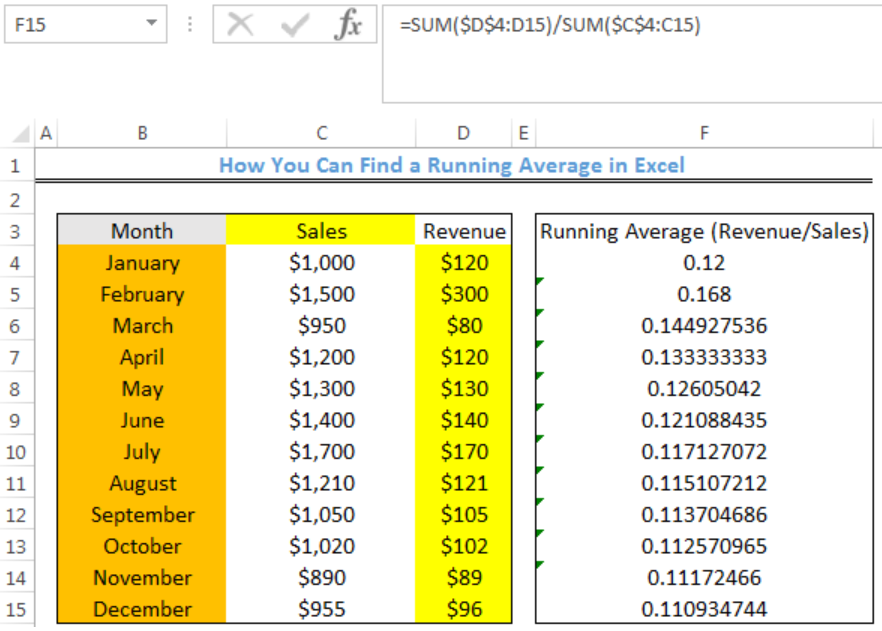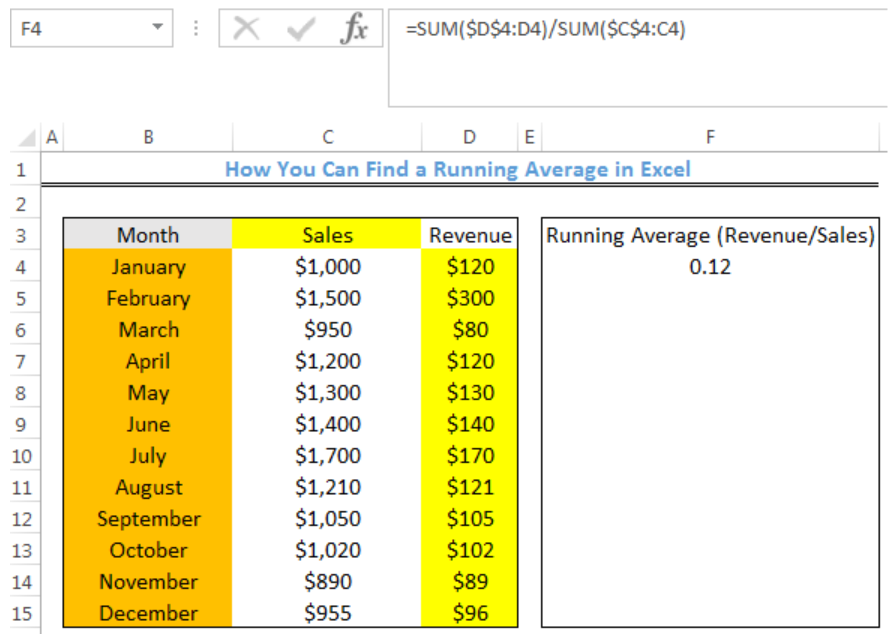Get instant live expert help with Excel or Google Sheets“My Excelchat expert helped me in less than 20 minutes, saving me what would have been 5 hours of work!”

#### Post your problem and you’ll get expert help in seconds.

Your message must be at least 40 characters
Our professional experts are available now. Your privacy is guaranteed.

# Here is How You Can Find a Running Average in Excel

We can use a formula that is based on the SUM function to calculate the RUNNING average for a set of values. The running average finds the average continuously from previous data points. The steps below will walk through the process.Figure 1- How to Find a Running Average in Excel

## Formula

`=SUM(\$D\$4:D4)/SUM(\$C\$4:C4)`

## Setting up the Data

We will calculate the running average as revenue divided by sales in figure 2.

• The months will be entered into Column B
• Column C and D contain the Sales and Revenue respectively
• The running average will be returned in Column FFigure 2 – Setting up the Data

## Running Average

• We will click on Cell F4
• We will insert the formula below into Cell F4
`=SUM(\$D\$4:D4)/SUM(\$C\$4:C4)`
• We will press the enter keyFigure 3- Running Average

• We will click on Cell F4 again
• We will double click on the fill handle tool which is the small plus sign you see at the bottom right of Cell F4. Select and drag down to copy the formula to Cell F15.Figure 4- Result of Running Average

## Explanation

If we consider Cell F6, the result is calculated in this manner:

`\$120+\$300+\$80/\$1000+\$1500+\$950`

`=0.144927536`

The next average is returned considering the next four values and so on.

## Instant Connection to an Expert through our Excelchat Service

Most of the time, the problem you will need to solve will be more complex than a simple application of a formula or function. If you want to save hours of research and frustration, try our live Excelchat service! Our Excel Experts are available 24/7 to answer any Excel question you may have. We guarantee a connection within 30 seconds and a customized solution within 20 minutes.

Are you still looking for help with the Average function? View our comprehensive round-up of Average function tutorials here.

Solution examplesI need a formula to pop at the upper left corner of a spreadsheet. If I enter the month "January," I want the column number sum of January =SUM(AB11:AB75) from another section on the same excel page to pop right below the "January" cell, and not display the formula expression, but see the \$100.
Solved by T. Q. in 40 minsCan't add (SUM) in imported numbers from bank account
Solved by F. C. in 40 minsI need a formula to combine D2 to D100 to add together a column of numbers, then take away the same amount on the same row when column E is filled. i.e. column D is a price of an item, so the formula must calculate the total, then when the item is sold an 'a' is marked next to the item in column E, the formula then must deduct this amount from the total
Solved by X. W. in 20 minsI would like to have a diagram in a new sheet, where the horizontal axis is the days, as they are in column DX. Each day shall show the sum of all unique leads of that day, and I would like to be able to check via a box of checkboxes, which facilities are shown, the facilities are in column BC.
Solved by I. A. in 45 minsI am working on a cash flow projection. Part of the projection includes sales commissions. Our sales guys earn a monthly draw and then commission on sales after a certain amount. For example they may earn a monthly salary of 12,500 and earn additional commission after their commission equals \$150,000. What I need excel to do is sum a column if the values in the preceeding columns are greater than \$150,000. I've tried using the sumif and the if function in excel and it's not working correctly either way. Any suggestions?
Solved by G. W. in 19 mins